<< Previous    1...   39  40    42  43  ...56    Next >>

#41 The first four enfolded polygons of the inner Tree of Life have 496 permutations of the rows of their 17 tetractys sectors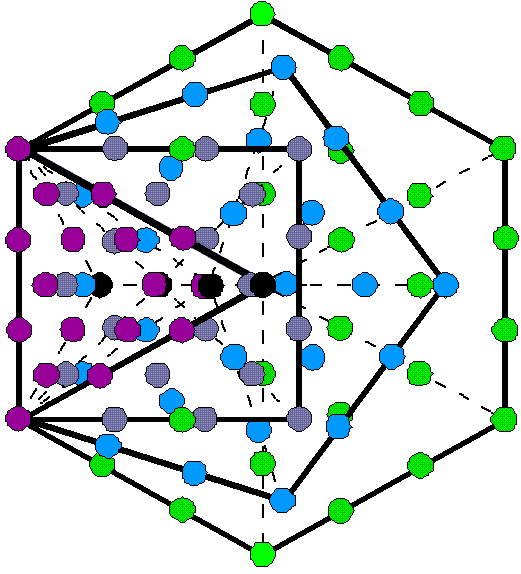The four rows of 1, 2, 3 & 4 yods in a tetractys have, respectively, (1! = 1) permutations, (2! = 2) permutations, (3! = 6) permutations & (4! = 24) permutations. The three lowest rows have (2+6+24=32) permutations (see also here). This means that 10 objects can be arranged in (1!×2!×3!×4! = 288) different tetractyses, where 288 = 11 + 22 + 33 + 44. When their 17 sectors are tetractyses, the first four enfolded polygons have 94 yods, that is, 90 yods outside their shared root edge. It was shown here that the yod populations of these polygons conform to the pattern of integers in the Lambda Tetractys, so that they constitute a holistic system. An n-gon with n tetractys sectors has 6n yods surrounding its centre. They are arranged in 3n rows of two or more yods that have 32n permutations. Its n sides, each with four yods, have (n×4!=24n) permutations and its second & third rows, each with, respectively, (2!=2) permutations and (3!=6) permutations, have (2n+6n=8n) permutations. Tabulated below are the numbers of permutations of yods in the root edge and in the 48 rows of the 17 tetractyses in the first four enfolded polygons:

Number of permutations of rows of yods in the first four enfolded polygons.

 Polygon Number of permutations on sides Number of permutations of 2nd & 3rd rows Total Root edge 24×1 = 24 − 24 Triangle 24×2 = 48 8×3 = 24 72 Square 24×3 = 72 8×4 = 32 104 Pentagon 24×4 = 96 8×5 = 40 136 Subtotal 240 96 336 Hexagon 24×5 = 120 8×5 = 40 160 Total 360 136 496

Their 49 rows of yods have 496 permutations. Remarkably, this is the number value of Malkuth, the last Sephirah of the Tree of Life: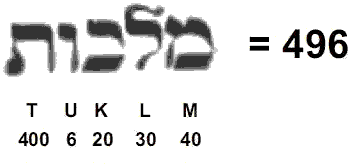and the dimension of SO(32) and E8×E8, the two symmetry groups that generate interactions between superstrings that are free of quantum anomalies (see here). As each permutation of two, three & four yods has its mirror-image counterpart, the 496 permutations consist of 248 permutations and their 248 mirror-images, where 248 is the dimension of E8. However, the actual division of the 496 permutations into two sets of 248 permutations may be more subtle than this, and we must analyse the contribution of each polygon in more detail. The first four enfolded polygons have 49 rows of yods with 496 permutations, showing how EL ChAI, the Godname of Yesod with number value 49, prescribes this number at the heart of superstring theory. Counting the sides of a polygon as its base, the apex of each tetractys sector is the centre of the polygon (shown in the diagram as a black yod). If we count this apex formally as a row, it has just one permutation, namely, itself. This means that, as well as the 496 permutations of the rows of 2, 3 & 4 yods in the 17 tetractyses, there are four permutations of just one yod, namely, the centres of the four polygons, so that the total number of permutations of the four rows of yods in each of the 17 tetractyses = 500 (=50×10). This shows how ELOHIM, the Godname of Binah with number value 50, prescribes the (49+4=53) rows of yods. YAH with number value 15 prescribes the first four enfolded polygons because they have 15 sides.

The table above indicates that the first three enfolded polygons have 240 permutations of the yods on their 10 sides (24 permutations per side) and 96 permutations of their 24 second & third rows — a total of 336 permutations. This chance-defying conjunction of the superstring dynamical parameters 240 & 496 and the structural parameter 336 of the UPA described paranormally by Annie Besant & C.W. Leadbeater is amazing. It constitutes powerful evidence for this basic unit of matter being a superstring. This is confirmed by the fact that the 14 sides of the first four enfolded polygons outside their shared root edge with 24 permutations of its four yods also have 336 permutations, so that:

24 + 336 + 136 = 496.

The seven sides of the square & pentagon outside the root edge have (72+96=168) permutations (84 permutations & their 84 mirror images) and the seven sides of the triangle & hexagon outside the root edge have (48+120=168) permutations (84 permutations & their 84 mirror images). The geometry of the first four enfolded polygons naturally allows the division 336 = 168 + 168 that is characteristic of holistic systems (see here). Its counterpart in the subquark superstring is the 336 turns in one revolution of a helical whorl of the UPA and the 168 turns in a half-revolution (84 in each quarter-revolution) (scroll down the left-hand column of the table here). Alternatively, the three sides of the triangle have 72 permutations and the seven sides of the square & pentagon have 168 permutations. The 72:168 division of the 240 permutations of the sides of the triangle, square & pentagon is a division that is characteristic of the parameter 240 embodied in all holistic systems; it corresponds to the 72 roots of E6, the rank-6 exceptional subgroup of E8, and the 168 other roots of E8. Given this remarkable correspondence between the 496 roots of E8×E8 and the 496 permutations of the 49 rows of yods in the first four enfolded polygons, the question arises: what eight permutations are the counterpart of the eight simple roots of each E8? If no natural counterparts exist in the geometry of the first four enfolded polygons, this would be evidence favouring SO(32) over E8×E8. However, analysis elsewhere in this website of other sacred geometries have certainly demonstrated their embodiment of the latter symmetry group, and so we should expect the geometry of the first four enfolded polygons to be able to generate these (8+8) permutations. Perhaps, however, one set of polygons corresponds to E8×E8 and its mirror image in the other half of the inner Tree of Life corresponds to SO(32)? Given that each side of a polygon generates 24 permutations of their four yods and that the second & third rows of yods in each tetractys generate eight permutations, it seems reasonable to infer that the eight simple roots in one E8 correspond to the eight permutations in the second & third rows in one of the 17 tetractyses and that the eight simple roots in the other E8 correspond to the eight permutations in the second & third rows in another tetractys. This leaves 120 permutations in the second & third rows of the remaining 15 tetractyses (120=8×15), as well as the (5×24=120) permutations of the yods in the five sides of the hexagon outside the root edge, making a total of 240 permutations. The two tetractyses should, intuitively speaking, be expected to belong to the simplest polygon — the triangle — because the simple roots of a Lie group are the most basic ones. The former 120 permutations comprise the eight permutations in the second & third rows of the third tetractys in the triangle and the 112 permutations of the second & third rows in the square, pentagon & hexagon (see table). The division: 240 = 112 + 8 +120 = 112 + 128 has its group counterpart in E8, as now explained. The E8 root system is a rank 8 root system containing 240 root vectors spanning R8. It is irreducible in the sense that it cannot be built from root systems of smaller rank. All the root vectors in E8 have the same length. It is convenient for a number of purposes to normalize them to have length √2. In the so-called even coordinate system E8 is given as the set of all vectors in R8 with length squared equal to 2 such that coordinates are either all integers or all half-integers and the sum of the coordinates is even. Explicitly, there are 112 roots with integer entries obtained from

(±1, ±1, 0, 0, 0, 0, 0, 0)

by taking an arbitrary combination of signs and an arbitrary permutation of coordinates, and 128 roots with half-integer entries obtained from

(±½, ±½, ±½, ±½, ±½, ±½, ±½, ±½)

by taking an even number of minus signs (or, equivalently, requiring that the sum of all the eight coordinates be even). There are 240 roots in all.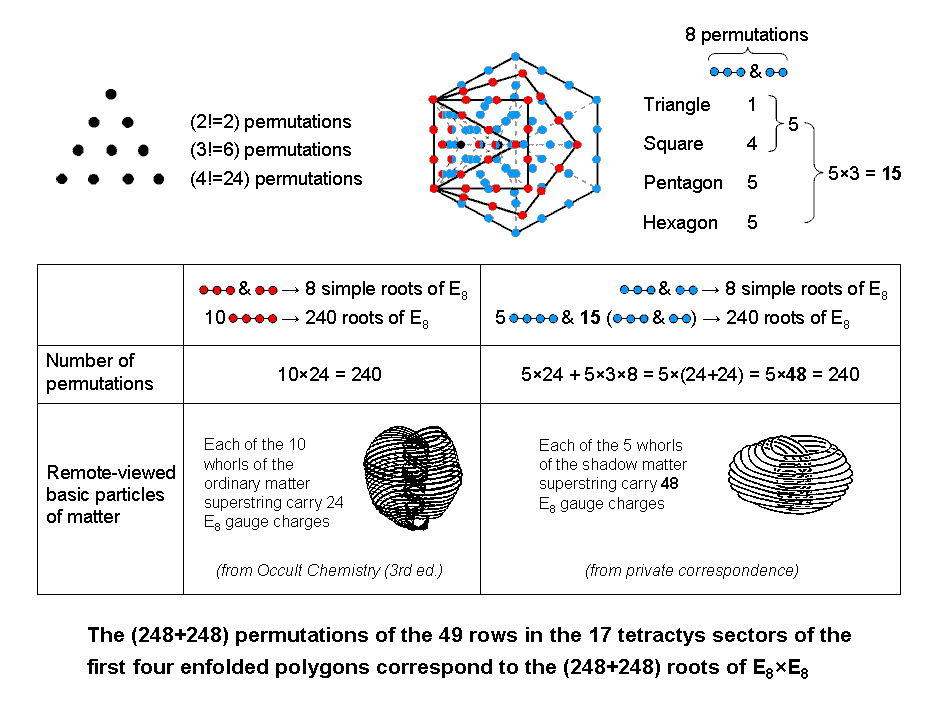The 28 red yods lining the 10 sides of the triangle, square & pentagon with black yods at their centres form 10 sets of 24 permutations of four yods corresponding to the 240 roots of E8. The tetractys sector with the root edge as its base has a row of two red yods and a row of three red yods (those yods shared with the two other sectors of the triangle are shown as half red and half blue). These two rows have eight permutations that correspond to the eight simple roots of E8. Hence, 12 rows of red yods have 248 permutations. The five sides of the hexagon outside the root edge have 14 blue yods and two red yods. They form five sets of 24 permutations of four yods, i.e., 120 permutations. One of the two remaining tetractys sectors in the triangle has two rows with eight permutations; they correspond to the eight simple roots of the second E8 group. The pair of rows in the other sector of the triangle, the four pairs of rows in the square, the five pairs of rows in the pentagon and the five pairs of rows in the hexagon constitute 15 pairs of rows of yods with (15×8=120) permutations. The number of permutations of the (5+2+2+8+10+10=37) rows of blue yods = 120 + 8 + 120 = 248.

Four rows have 16 permutations corresponding to the 16 simple roots of the two similar E8 groups and 45 rows have 480 permutations corresponding to their 480 roots. The 10 sides of the first three enfolded polygons generate 240 permutations of red yods (24 per side). There remain five sides of the hexagon and three sets of five pairs of rows, i.e., 35 rows of blue yods, with 240 permutations. They create five sets of seven rows (one side with 24 permutations & three pairs of rows with (3×8=24) permutations. In other words, the 240 roots in one E8 correspond to 10 sets of 24 permutations and the 240 roots in the other E8 correspond to five sets of (24+24=48) permutations. Article 53 demonstrates the 10-fold division of the holistic parameter 240 embodied in five sacred geometries. It manifests in the subquark state of the E8×E8 heterotic superstring paranormally described over a century ago by Annie Besant & C.W. Leadbeater as the 10 whorls of their UPA. 24 gauge charges of E8 are spread along each whorl. It was shown here that the seven separate Type B polygons of the inner Tree of Life are composed of 240 geometrical elements surrounding the centres of the triangle, square, pentagon & dodecagon and 240 geometrical elements surrounding the centres of the hexagon, octagon & decagon. The former polygons form 10 sets of 24 elements and the latter polygons form five sets of 48. It was proposed that the former correspond to the 10 whorls of the UPA and that the latter correspond to the five whorls reported by another remote viewer (private communication) to constitute the superstring making up shadow matter whose forces are described by the second E8 group. We now find support for this interpretation in the five-fold pattern of distribution of the 240 permutations of the 37 rows of blue yods. The 10 sides of the triangle, square & pentagon determine the 10 whorls of the superstring of ordinary matter and the five sides of the hexagon outside the root edge, the five sectors of the pentagon and the five remaining sectors of the square & triangle determine the five whorls of the superstring of shadow matter.

There are 360 permutations of the yods in the 15 sides and 136 permutations of the 34 second & third rows. 496 is the 31st triangular number:

1 + 2 + 3 + ... + 31 = 496,

and 136 is the 16th triangular number:

1 + 2 + 3 + ... + 16 = 136.

A pentagon whose five sectors are tetractyses contains 31 yods. 30 yods surround its centre. 31 is the number value of EL (אל), the Godname of Chesed. The value 1 of the letter aleph (א) denotes the centre of the pentagon and the value 30 of lamed (ל) denotes the 30 yods. 15 black yods lie on the sides of the pentagon, inside which are 16 red yods: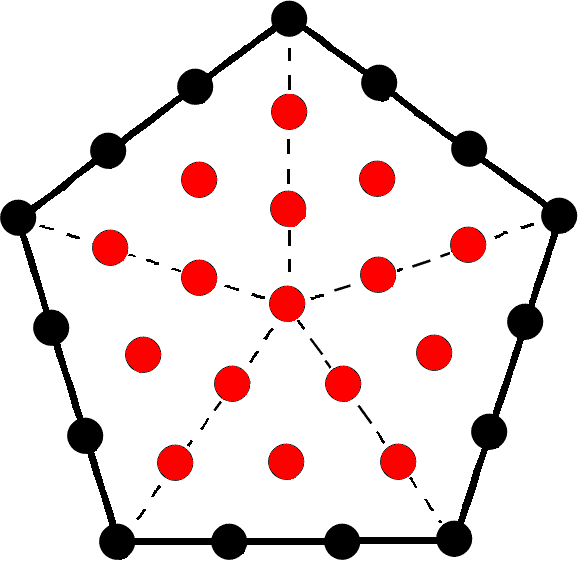Assigning the integers 1–31 to the 31 yods, starting with 1 assigned to the central yod, the first 16 integers inside the pentagon add up to 136 and the next 15 integers 17–31 assigned to yods on its sides add up to 360. This division is the counterpart of the 496 permutations (see the last row in the table above). The interior of the pentagon generates the number of permutations of the 34 second & third rows and its boundary generates the number of permutations of the 15 sides of the first four enfolded polygons. Displayed here is a remarkable harmony of number and sacred geometry co-existing with an equally remarkable conjunction of theoretical physics (namely, the number 496 at the heart of superstring theory) and the paranormal observations of Besant & Leadbeater (namely, the number 336, which is the number of circular turns in one revolution of each helical whorl in a UPA).

The 90 yods outside the root edge of the first four enfolded polygons comprise 13 corners of 17 tetractyses and 77 hexagonal yods. The first (4+4) enfolded polygons have 34 tetractyses with 184 yods made up of (13+13=26) corners outside the root edge and 156 hexagonal yods. 26 is the number value of YAHWEH, the Godname of Chokmah (Hebrew: YHVH), and 156 is the sum of the values of all combinations of the Hebrew letters of YHVH:

YHVH = 26.

Y = 10, H = 5, V = 6.

Y + H + V = 21;

YH + YV + VH + HH = 52;

YHV + YHH + HVH = 57;

YHVH = 26.

Total = 156.

(17+17=34) hexagonal yods are at the centres of the 34 tetractyses and (184−34=150=15×10) yods line their 61 sides, where 15 is the number value of YAH, the older Godname of Chokmah. (150−4=146) yods line the 34 tetractyses outside the root edge. 73 yods outside the root edge line the 17 tetractyses in each set of four enfolded polygons. 73 is the number value of Chokmah. The first (4+4) enfolded Type A polygons have 34 sectors with 61 sides and 28 corners, i.e., 123 geometrical elements. This is the tenth Lucas number L10, i.e., the tenth member of the Lucas series after the first one, L0 = 2:

2, 1, 3, 4, 7, 11, 18, 29, 47, 76, 123, 199, 322, ...

so that it is determined by the Decad (10). In terms of the Golden Ratio Φ = (√5+1)/2 = 1.6180339887...., the nth Lucas number Ln = Φn + (−Φ)−n, so that

123 = L10 = Φ10 + Φ−10 = Φ10 + φ10,

where φ = Φ−1 = Φ−1 = (√5−1)/2 = 0.6180339887... .This is how the Golden Ratio and the Decad express the geometrical composition of the first (4+4) enfolded Type A polygons, each half of which embodies the third perfect number 496*:

496 = 1 + 2 + 4 + 8 + 16 + 31 + 62 + 124 + 248

at the heart of superstring theory! The second perfect number 28:

28 = 1 + 2 + 4 + 7 + 14

also appears in them as the number of corners of their 34 sectors, whilst the first perfect number 6:

6 = 1 + 2 + 3

is the number of corners of the hexagon, which is the fourth polygon.

The 3-tree (the lowest three Trees of four or more overlapping Trees of Life) also possesses 123 points, lines & triangles: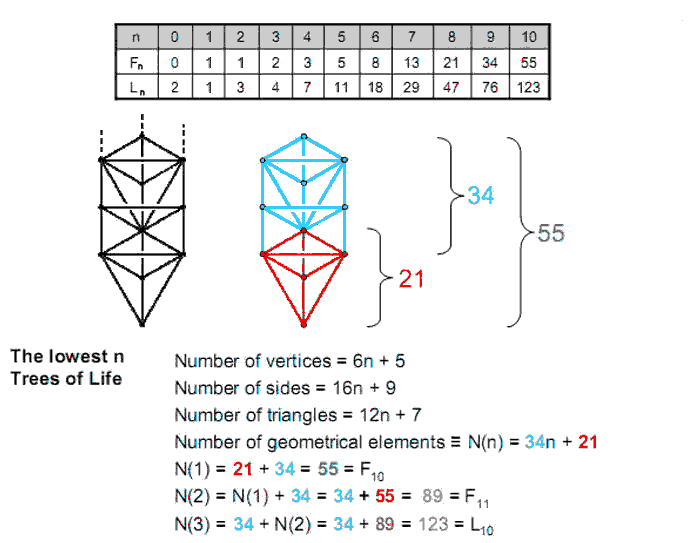(Fn is the nth Fibonacci number. They are coloured in the diagram shown above). Article 32 explains the significance of the 3-tree and its inner form as the Tree of Life map of the human skeleton and the 14 acupuncture meridians.

120 geometrical elements are outside the root edge of the first (4+4) enfolded Type A polygons, where

120 = 22 + 42 + 62 + 82.

The Decad also determines this number because it is the sum of the first ten odd integers after 1:

120 = 121 − 1 = 112 − 1 = 3 + 5 + 7 + 9 + 11 + 13 + 15 + 17 + 19 + 21.

See Article 8 for a discussion of the properties of the first four polygons — both separate and enfolded — and their prescription by the Godnames of the 10 Sephiroth. The holistic nature of the first (4+4) enfolded polygons is proved in Article 48.

The Tree of Life pattern of the 248 permutations and their 248 mirror images
The 496 permutations of the 2nd, 3rd & 4th rows of yods in the 17 tetractyses making up the first four enfolded polygons comprise 248 permutations and their 248 mirror images. The contributions by the polygons to either set of permutations are tabulated below:

 Polygon Number of permutations on sides Number of permutations of 2nd & 3rd rows Total Root edge 12×1 = 12 − 12 Triangle 12×2 = 24 4×3 = 12 36 Square 12×3 = 36 4×4 = 16 52 Pentagon 12×4 = 48 4×5 = 20 68 Subtotal 12 + 108 = 120 48 168 Hexagon 12×5 = 60 4×5 = 20 80 Total 12 + 168 = 180 68 248

The root edge contributes 12 permutations, the 14 external sides of the polygons contribute (14×12=168) permutations and the 34 2nd & 3rd rows of the 17 tetractyses contribute 68 permutations. There are 168 permutations of the yods shaping the first four enfolded polygons by lining their sides outside the root edge and (12+68=80) permutations of the yods either on the root edge or in rows inside the boundaries of the polygons. This 80:168 division is characteristic of holistic systems embodying the holistic parameter 248 (see here & here). The triangle, square & pentagon have 48 permutations of their 24 2nd & 3rd rows, leaving (12+20=32) permutations in either the root edge or the 10 2nd & 3rd rows of the five tetractyses in the hexagon. This 48:32 division of the holistic parameter 80 is characteristic of holistic systems. For example, the 80 yods in the 1-tree comprise the 48 yods up to Chesed and the 32 yods above it (see here). Amazingly, the 48:32:168 division of the 248 permutations and their 248 mirror images conforms to the yod composition of the 248 yods up to the 31st SL, which is Chesed of the fifth Tree of Life prescribed by EL. This amounts to confirmation of the superstring significance of these 248 permutations. It is highly improbable that such a natural geometrical counterpart in the first four polygons to the yod composition up to the 31st SL could occur by chance. The 15 rows of yods in the five sectors of the hexagon that are not shared with the triangle also contribute 80 permutations to the 168 permutations provided by the triangle, square & pentagon. However, this cannot be the correct counterpart of the 80 roots of E8 that are either simple roots or roots of its exceptional subgroup E6 because it is counter-intuitive to make the eight simple roots of E8 analogous to the eight permutations of the yods in the rows of 2 & 3 in two of the five tetractyses of the hexagon (notice that each tetractys contributes four such permutations), there not being two tetractys sectors in this polygon that differ in some way to the others. On the other hand, the 8:72 split of the 80 roots does have a natural counterpart in the 80 permutations of yods that are either on the root edge or in rows of 2 & 3 because the triangle has two tetractys sectors unshared with the root edge, each of which can provide four permutations of its 2nd & 3rd rows, that is, eight permutations that correspond to the eight simple roots. So it seems intuitively more correct to associate the 80:168 division with what was described earlier than with the difference between the hexagon with 80 permutations and the triangle, square & pentagon with 168 permutations. Admittedly, one would like a more rigorous reason than this intuitive argument. But that must await a full explanation of what the 248 permutations of the 2nd, 3rd & 4th rows of the 17 tetractyses in the first four enfolded polygons mean vis-à-vis the root composition of E8. What is vital to notice here is that, if we do not divide the set of permutations generated by each row of yods into two equal subsets, one containing the mirror images of the others, then we find that the 49 rows of yods have 496 permutations; this is the dimension of SO(32). When, however, we divide the 32 permutations provided by each tetractys into 16 permutations and their mirror images, then we find that there are (248+248=496) permutations; this is the dimension of E8×E8. In other words, the two symmetry groups at the heart of superstring theory have their separate counterparts in the permutational potential provided by the tetractys sectors of the first four enfolded polygons, which constitute a holistic system in themselves. The analogy explains why two distinct symmetry groups with the same dimension account for superstring forces. One (the counterpart of SO(32)) makes no distinction between left and right with regard to any permutation; the other (the counterpart of E8×E8) does. It should be noted that each symmetry group is equally fundamental. It is not that SO(32) is any more fundamental than E8×E8, for the mirror permutations always exist, whatever the objects symbolised by the yods, and no additional assumption of mirror symmetry has to be made in order to create a distinction between two sets of permutations that would cause the emergence of E8×E8 from SO(32).

* A perfect number is a positive integer that is the sum of its positive divisors (including 1) other than itself.

 << Previous    1...   39  40    42  43  ...56    Next >>

Home